## 任务定义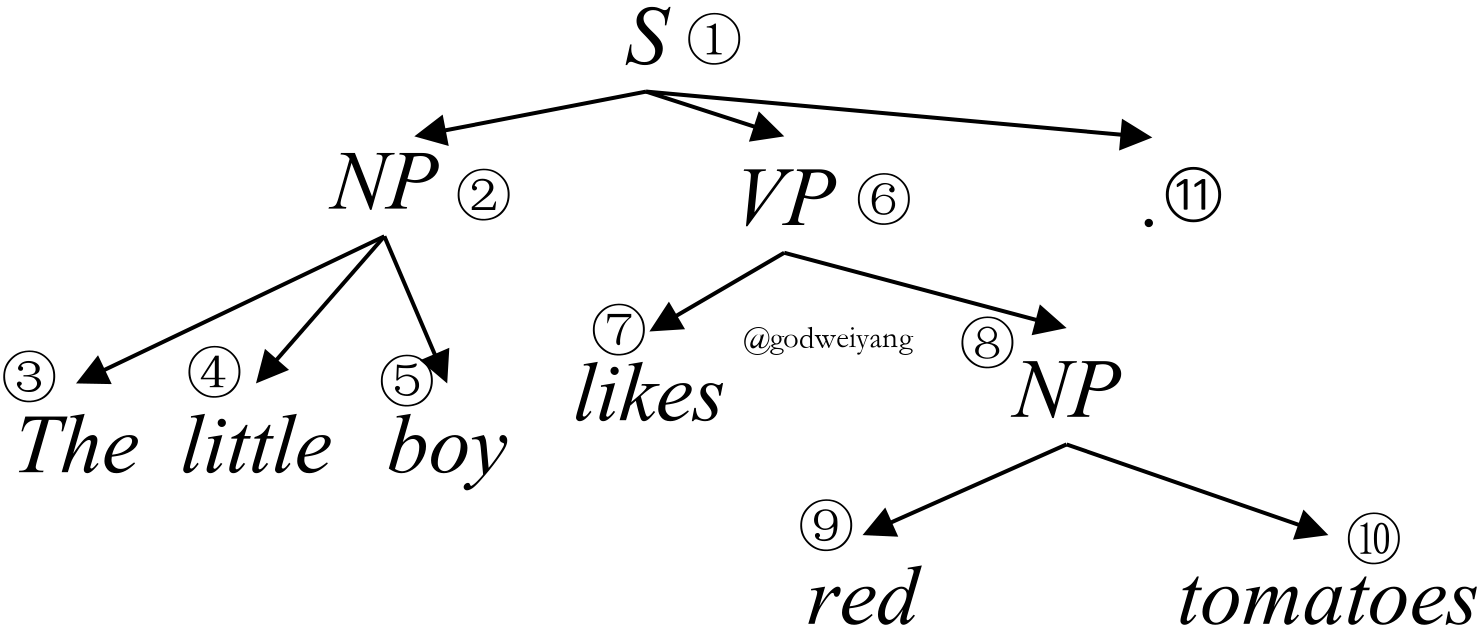$$T = \{(i, j, \ell) | (i, j, \ell) \in T\}.$$

## 编码模型

$$x_i = [e_i; p_i; c_i].$$

### LSTM编码

$$s_{ij} = [\mathord{\buildrel{\hbox{\scriptscriptstyle\rightarrow}}\over{h}}_j - \mathord{\buildrel{\hbox{\scriptscriptstyle\rightarrow}}\over{h}}_i; \mathord{\buildrel{\hbox{\scriptscriptstyle\leftarrow}}\over{h}}_i - \mathord{\buildrel{\hbox{\scriptscriptstyle\leftarrow}}\over{h}}_j].$$

### Transformer编码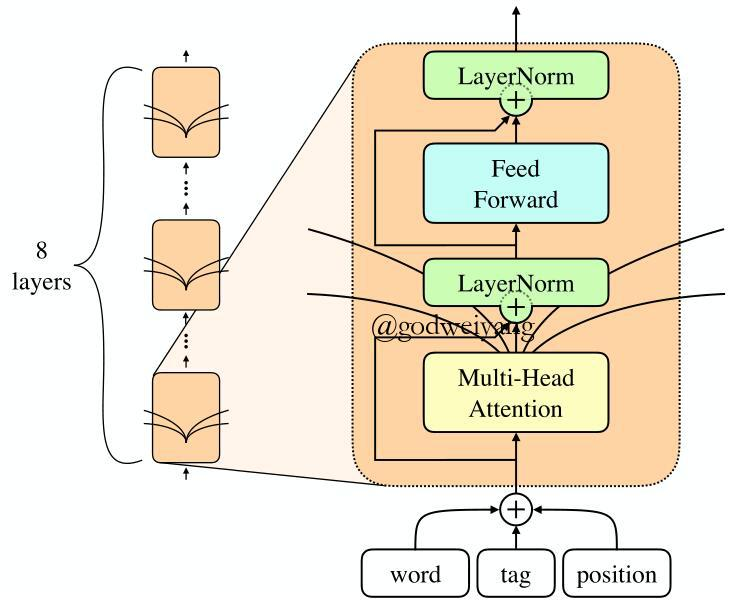Transformer的输入有三个，查询（query）向量矩阵$Q$，键（key）向量矩阵$K$和值（value）向量矩阵$V$。

$${\rm Attention}(Q, K, V) = {\rm softmax}(\frac{QK^{\top}}{\sqrt{d_k}})V.$$

### 递归神经网络编码

$$s_{ij} = f({\bf W}[s_{ik};s_{kj}]+{\bf b}),$$

### 得分计算

\begin{aligned} s_{\rm{label}}(i, j, \ell) & = {\bf v}_{\ell}^{\top}f({\bf W}_{\ell}^2f({\bf W}_{\ell}^1s_{ij} + {\bf b}_{\ell}^1) + {\bf b}_{\ell}^2), \\ s_{\rm{span}}(i, j) & = {\bf v}_s^{\top}f({\bf W}_{s}^2f({\bf W}_{s}^1s_{ij} + {\bf b}_{s}^1) + {\bf b}_{s}^2), \end{aligned} \tag{1}

$$s_{\rm tree}(T) = \sum\limits_{(i, j, \ell) \in T}{s_{\rm{label}}(i, j, \ell) + s_{\rm{span}}(i, j)}.$$

## 基于转移系统的解码算法

### 自底向上的转移系统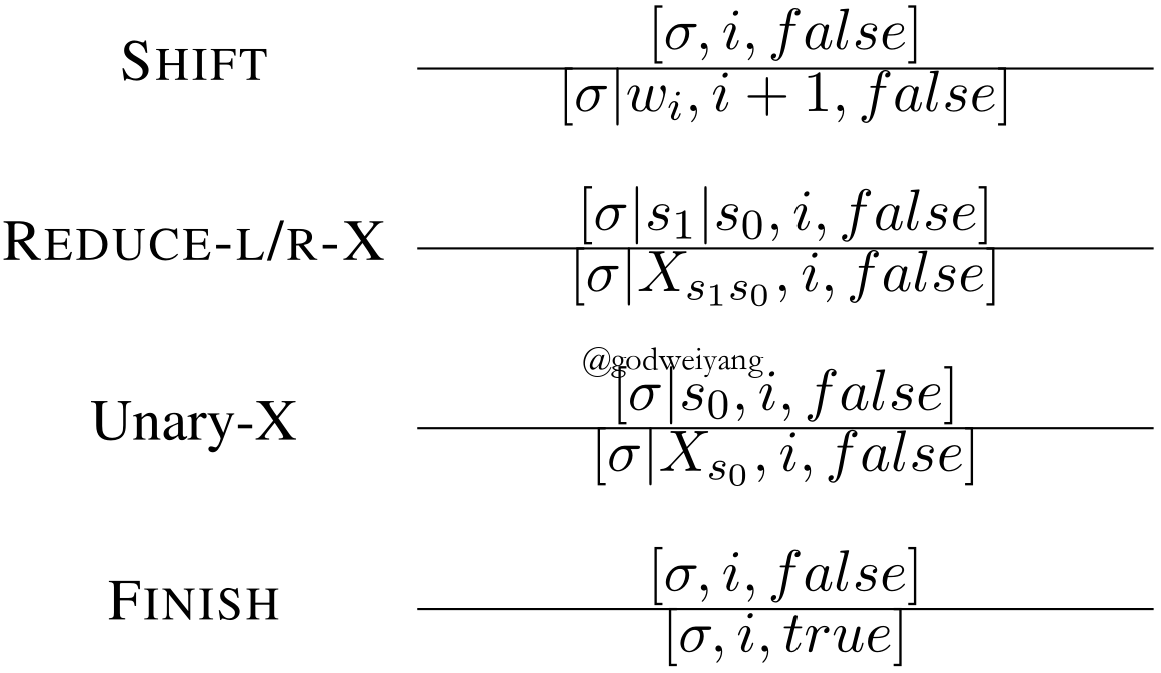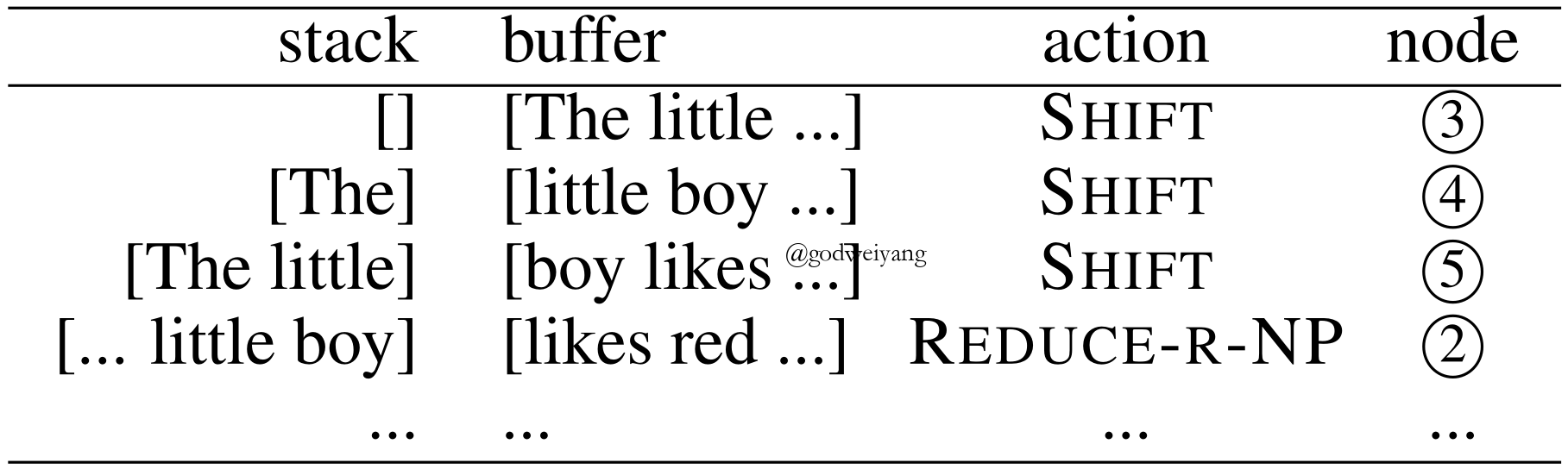### 自顶向下的转移系统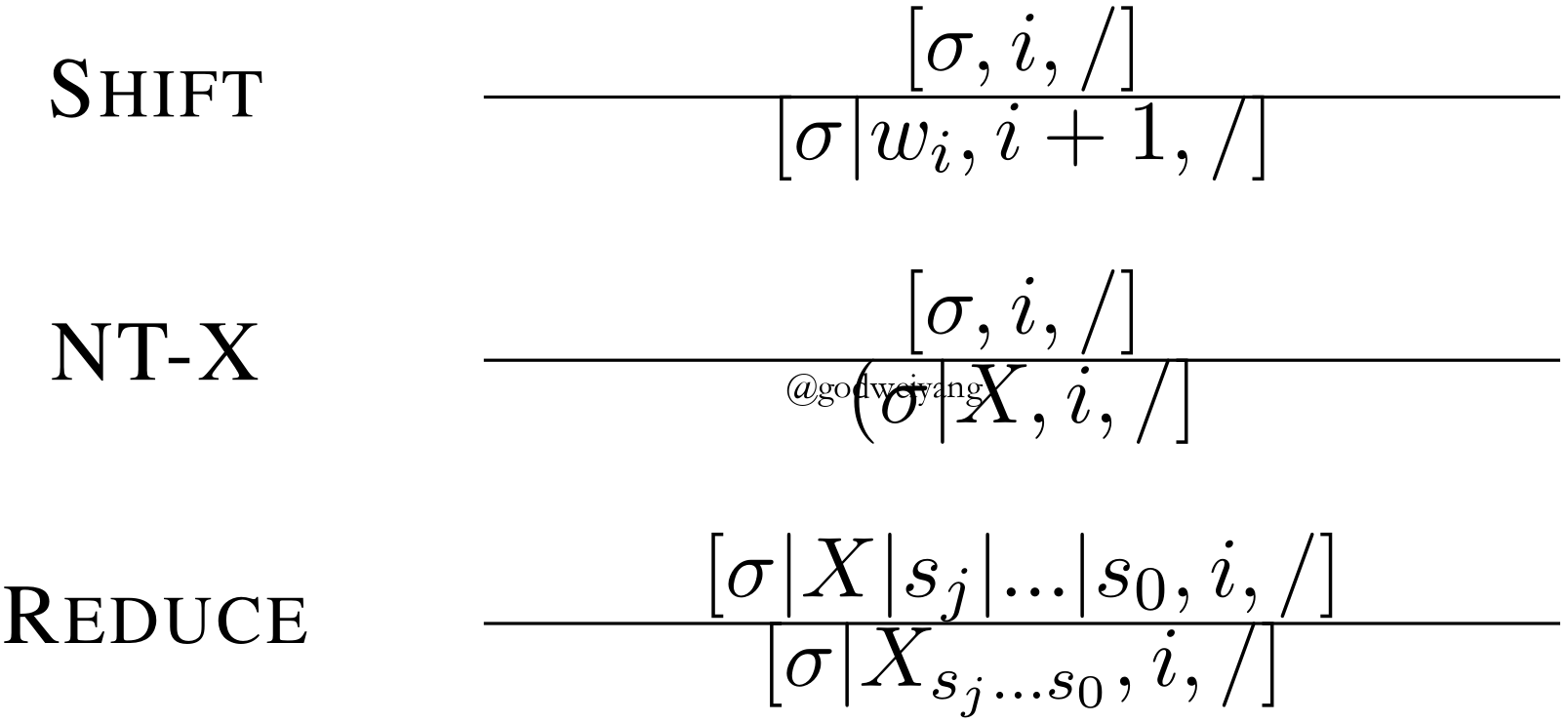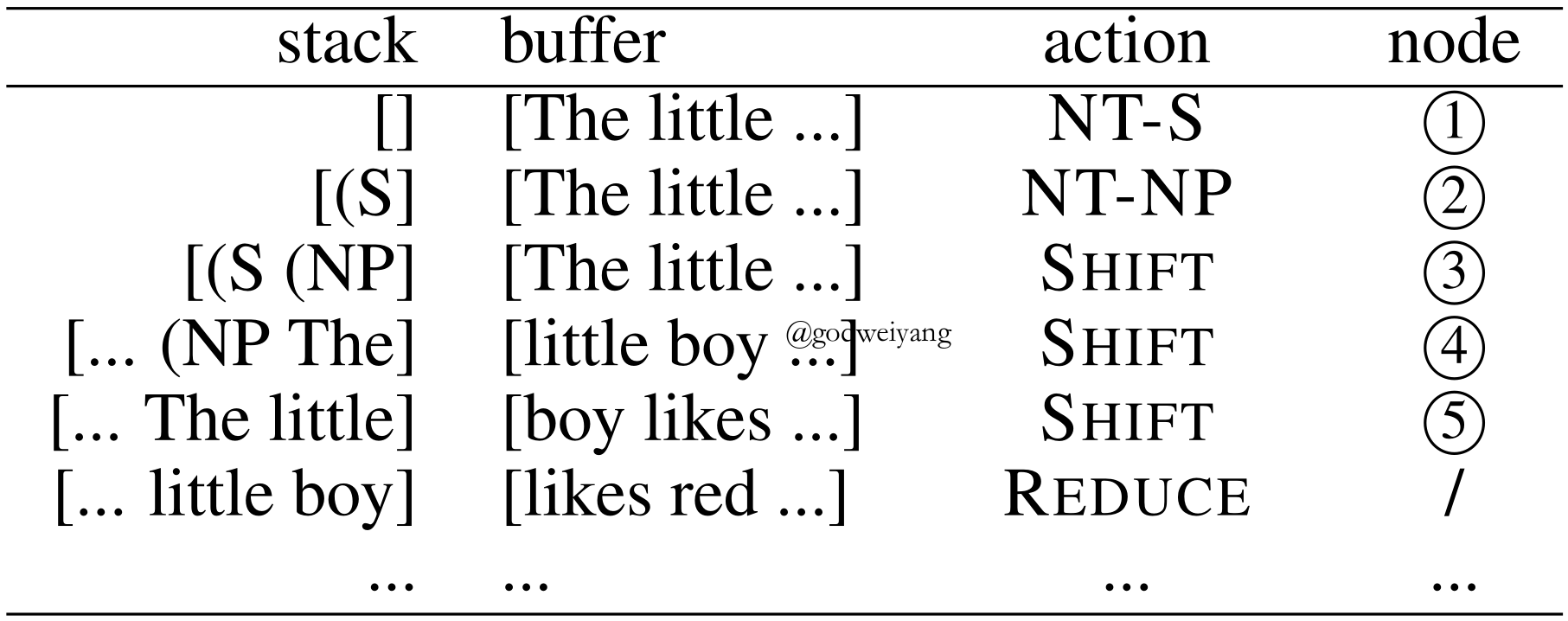### 基于中序遍历的转移系统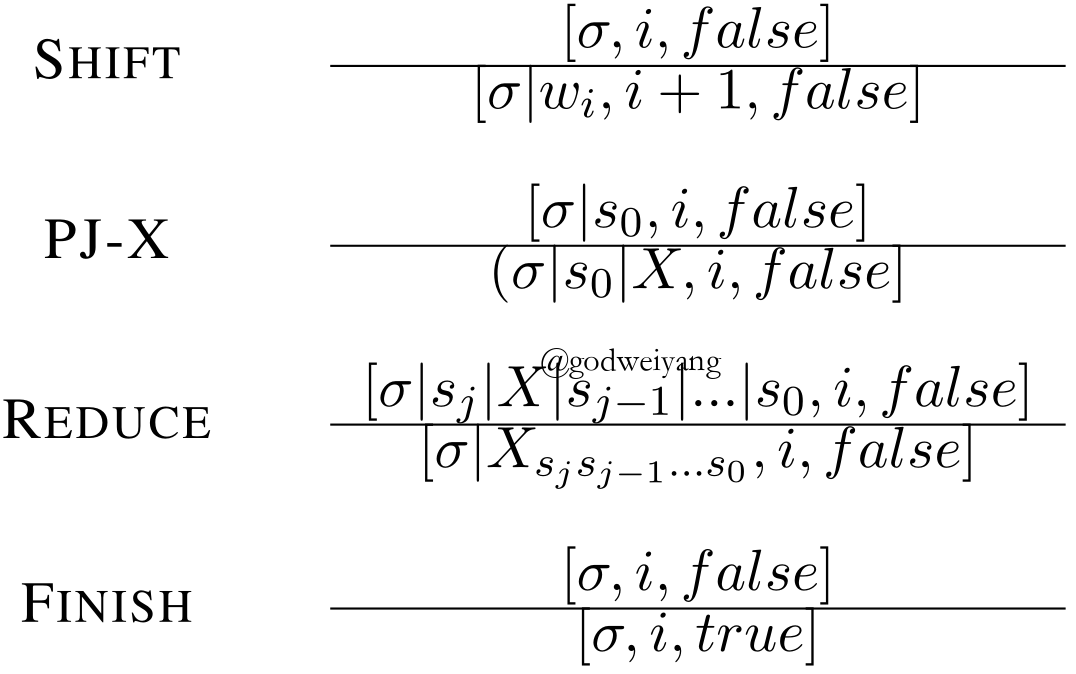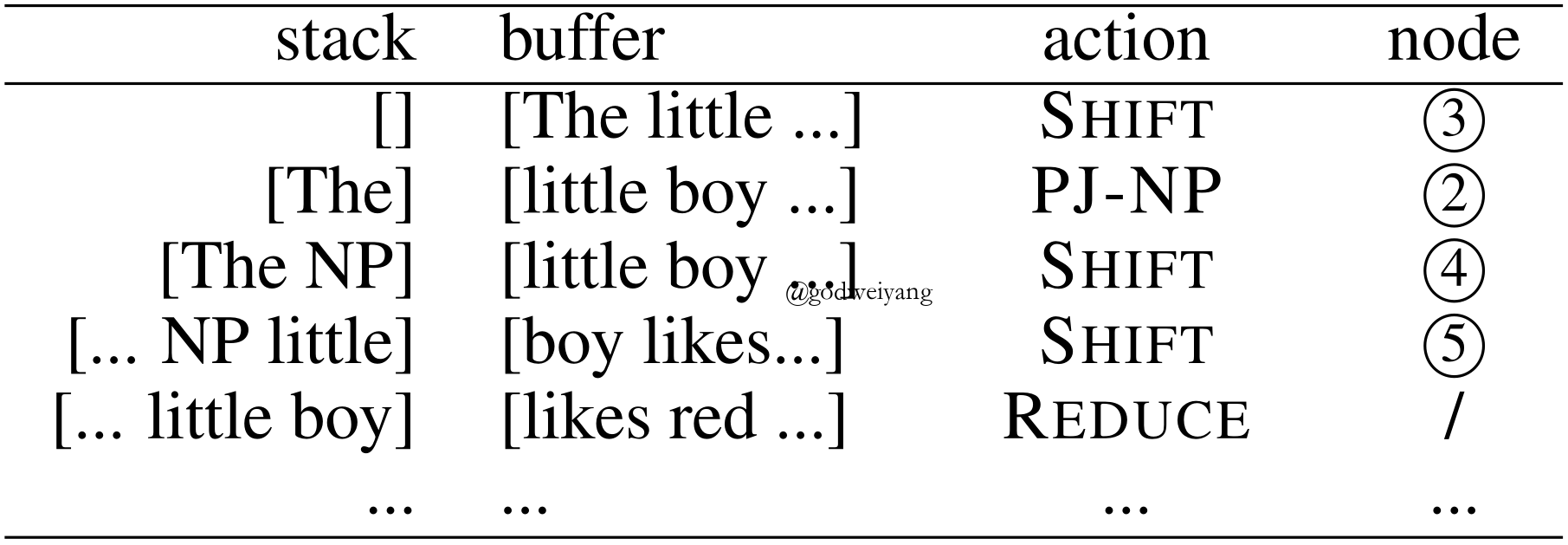### 生成模型

\begin{aligned} p(x) & = \sum\nolimits_{y \in \mathcal{Y}(x)} {p(x,y)} \\ & = \sum\nolimits_{y \in \mathcal{Y}(x)} {q(y|x)w(x,y)} \\ & = {\mathbb {E}_{q(y|x)}}w(x,y), \end{aligned}

$${y^{(i)}} \sim q(y|x),i = 1,2, \ldots ,N.$$

\begin{aligned} p(x) & = {\mathbb{E}_{q(y|x)}}w(x,y) \\ & \mathop \approx \limits^{\rm MC} \frac{1}{N}\sum\limits_{i = 1}^N {w(x,{y^{(i)}})}. \end{aligned}

## 基于动态规划的解码算法及其变体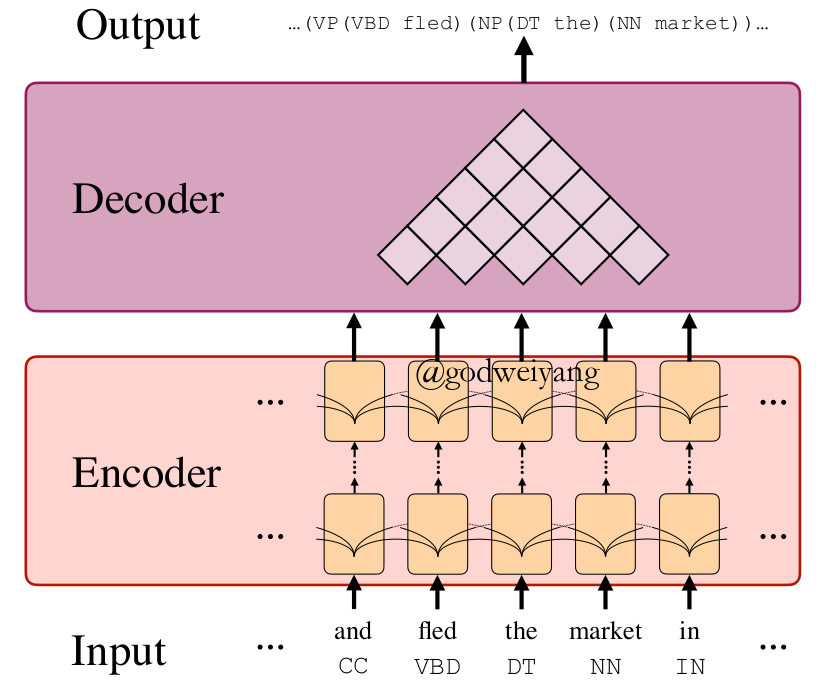### 动态规划解码

\begin{aligned} s_{\rm split}(i, j, k) & = s_{\rm label}(i, j, \hat \ell_{ij}) \\ & + s_{\rm label}(i, k, \hat \ell_{ik}) + s_{\rm label}(k, j, \hat \ell_{kj}). \end{aligned}

### 自顶向下贪心解码

$$\begin{array}{l} \hat \ell = \mathop {\arg \max }\limits_l [{s_{\rm label}}(i,j,\ell)] \\ \hat k = \mathop {\arg \max }\limits_k [{s_{\rm split}}(i,j,k)]. \end{array}$$

## 基于序列到序列的解码算法

### 基于括号表达式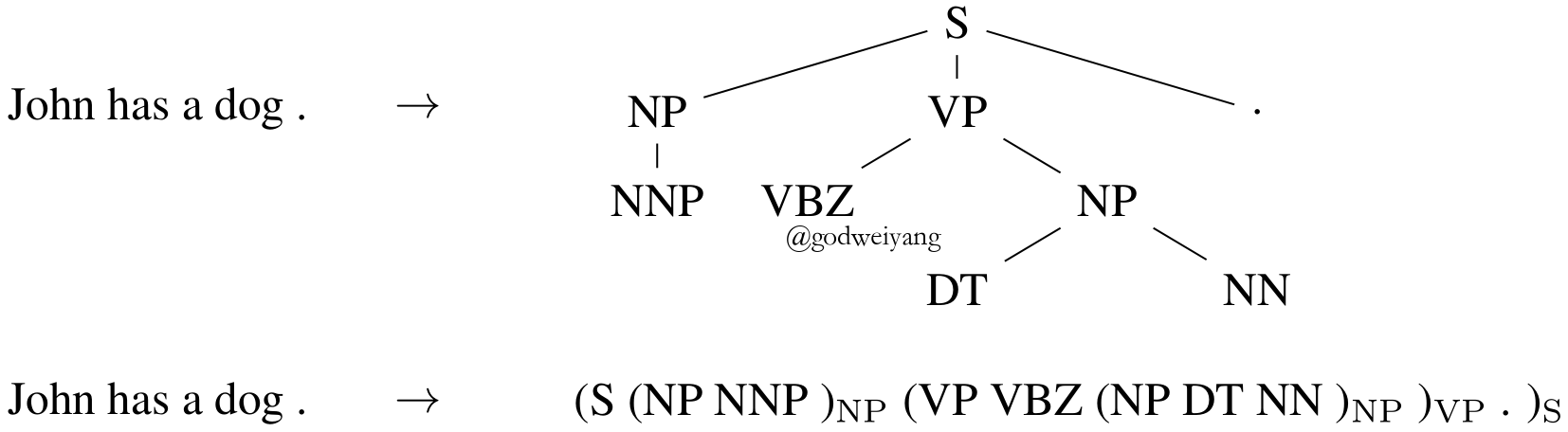### 基于句法距离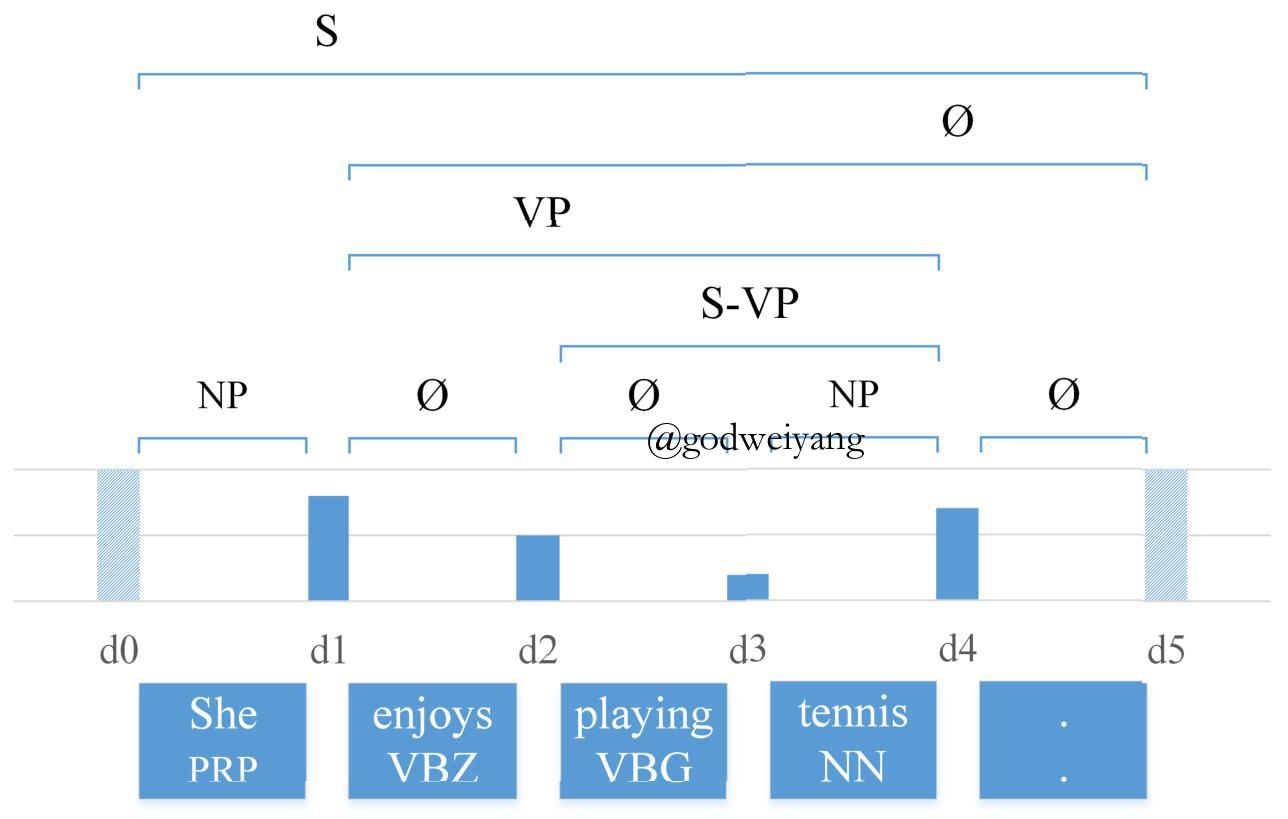## 若干改进

### 策略梯度

$$\mathcal{R}(\theta ) = \sum\limits_{i = 1}^N {\sum\limits_y {p(y|{x^{(i)}};\theta )\Delta (y,{y^{(i)}})} },$$

$$\nabla \mathcal{R}(\theta ) \approx \sum\limits_{i = 1}^N {\sum\limits_{y \in \mathcal{Y}({x^{(i)}})} {\Delta (y,{y^{(i)}})\nabla \log p(y|{x^{(i)}};\theta )} },$$

## 实验

### 实验结果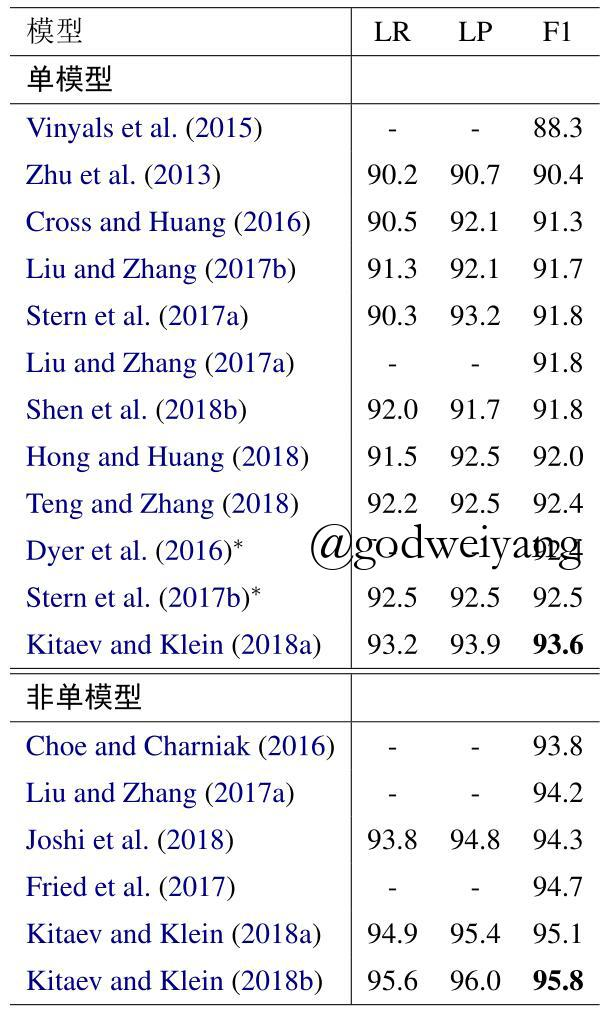## 参考文献

• Attention is All you Need
• Transition-based Neural Constituent Parsing
• Span-Based Constituency Parsing with a Structure-Label System and Provably Optimal Dynamic Oracles
• In-Order Transition-based Constituent Parsing
• A Minimal Span-Based Neural Constituency Parser
• Constituency Parsing with a Self-Attentive Encoder
• Straight to the Tree: Constituency Parsing with Neural Syntactic Distance
• Constituent Parsing as Sequence Labeling
• Grammar as a Foreign Language
• Two Local Models for Neural Constituent Parsing
• Long Short-Term Memory Over Tree Structures
• Dependencies vs. Constituents for Tree-Based Alignment
• A tutorial on particle filtering and smoothing: Fifteen years later
• Programming languages and their compilers: Preliminary notes
• Parsing with Compositional Vector Grammars
• Policy Gradient as a Proxy for Dynamic Oracles in Constituency Parsing
• Multilingual Constituency Parsing with Self-Attention and Pre-Training
• Fast and Accurate Shift-Reduce Constituent Parsing
• Shift-Reduce Constituent Parsing with Neural Lookahead Features
• Linear-time Constituency Parsing with RNNs and Dynamic Programming
• Recurrent Neural Network Grammars
• Effective Inference for Generative Neural Parsing
• Parsing as Language Modeling
• Extending a Parser to Distant Domains Using a Few Dozen Partially Annotated Examples
• Improving Neural Parsing by Disentangling Model Combination and Reranking Effects
• Neural Language Modeling by Jointly Learning Syntax and Lexicon
• Gaussian Mixture Latent Vector Grammars

转载规则

《成分句法分析综述（第二版）》 韦阳 采用 知识共享署名 4.0 国际许可协议 进行许可。
上一篇二零一九年终总结

2019-12-13微信聊天记录导出为电脑txt文件教程

2019-08-09
目录